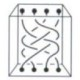# Why is a negative divided by a negative a positive?The Number Warrior

So there’s a whole lot of posts, including one from this very blog, which give intuitive explanations of why a negative times a negative is a positive.I haven’t seen nearly as much material for a negative divided by a negative. One can certainly appeal to the inverse — since $latex 1 \times -1 = -1$, $latex \frac{-1}{-1} = 1$. Google searching leads to answers like that, but I’ve found nothing like the multiplication picture above.

Can anyone explain directly, at an intuitive level, why a negative divided by a negative is a positive? Or is the only way to do it to refer to multiplication?

View original post## Author: mathtuition88

https://mathtuition88.com/

This site uses Akismet to reduce spam. Learn how your comment data is processed.